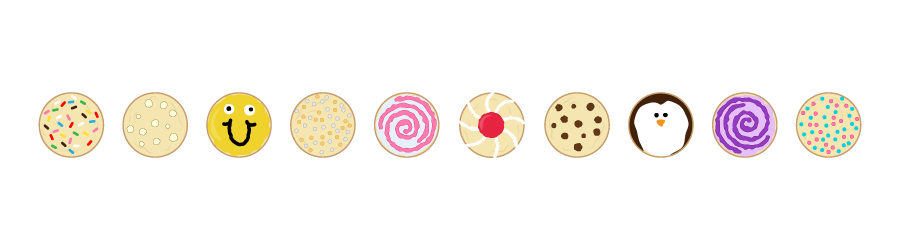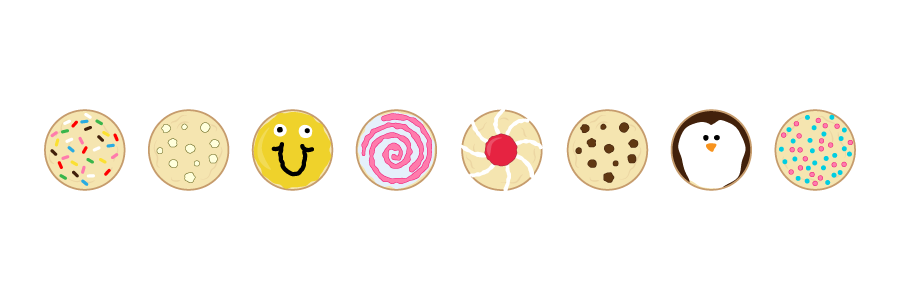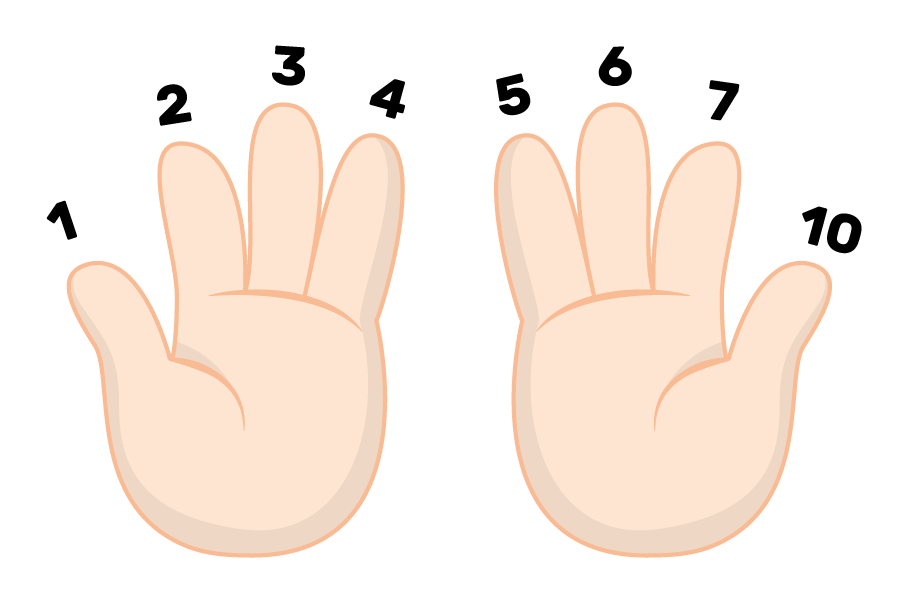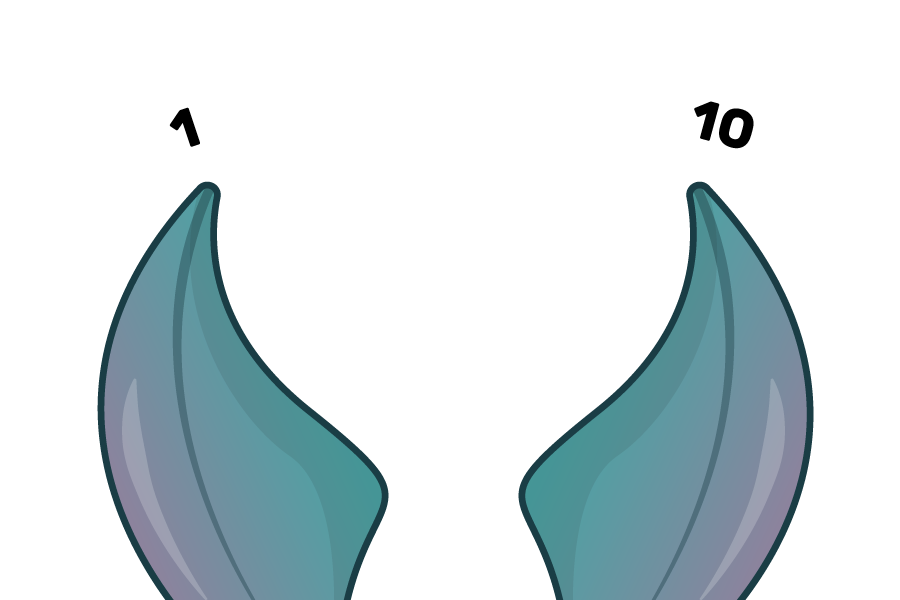## Want to keep learning?

This content is taken from the Raspberry Pi Foundation & National Centre for Computing Education's online course, Representing Data with Images and Sound: Bringing Data to Life. Join the course to learn more.
1.4

# Reviewing Binary

To understand how computers bring data to life, you need to understand the basic number system that computers use: binary. This section gives an overview the binary number system and how to convert between binary and our standard number system. For a more comprehensive look at binary in computing, try our How Computers Work course.

### Number systems

The number system we normally use is the base 10 or decimal system, also called denary. This system is based on the number ten, which is a very important number for us humans. Because we have ten fingers, when someone tells us: “I have ten cookies”, we will immediately assume this amount of cookies because we are used to counting with ten fingers:However, some cartoon characters have only eight fingers. If we told them that we had ten cookies, they might think that we had this many cookies:These cartoon characters would probably have their own number system that isn’t based on the number ten, but the number of fingers they have: eight. We would call this an octal number system, or base 8. This is how they might count:What number system would fish have?

If you guessed base 2 or binary, you’re correct! Because Nemo and Dory are fish and have two main fins, if we told them we have ten cookies, this is what they might think:And this is how they might count the cookies:

• 1
• 10

This is similar to a computer’s number system because, as you learned in the previous step, computers are made up of billions of switches, and switches only have two states: on and off.

Here’s a table summarising how the binary number system compares to the decimal system when looking at powers of two. The binary numbers look like they are very large quantities, but 100000000 in binary is the same quantity as 256 in denary, it just looks longer.

Power of two Denary Binary
2.00E+00 1 1
2.00E+01 2 10
2.00E+02 4 100
2.00E+03 8 1000
2.00E+04 16 10000
2.00E+05 32 100000
2.00E+06 64 1000000
2.00E+07 128 10000000
2.00E+08 256 100000000

Have a look at the denary column to become familiar with these numbers, because we use them to convert from denary to binary and vice versa. We will now go into more detail about this, using a binary magic trick as an introduction.

### Binary magic trick

When I worked as a teacher, I enjoyed introducing my students to binary conversion by doing a magic trick. Here’s how it works.

Col A Col B Col C Col D Col E
16 8 4 2 1
17 9 5 3 3
18 10 6 6 5
19 11 7 7 7
20 12 12 10 9
21 13 13 11 11
22 14 14 14 13
23 15 15 15 15
24 24 20 18 17
25 25 21 19 19
26 26 22 22 21
27 27 23 23 23
28 28 28 26 25
29 29 29 27 27
30 30 30 30 29
31 31 31 31 31
• Ask the person being tricked to pick a number from 1 to 31 but not tell you what it is (as an example, let’s say they pick 6)
• Get them to tell you which columns their number is in in the table (C and D)
• Tell them what their number is by using the top row of the grid and adding up the numbers right below the corresponding columns (6 appears in columns C and D, so the calculation is 4 + 2 = 6)

To make sure the trick goes smoothly, you can memorise the numbers below the column headings (16, 8, 4, 2, 1), but if you forget them, you can start from 1 on the right and multiply the number by 2 to get the next number to the left.

The trick prepares you for converting binary to denary, and helps you remember how it is done.

### Denary to binary

Computers, because they are built of millions of tiny circuits, use binary and only really understand two things: on and off, corresponding to 1 and 0. Binary numbers increase in size according to our number system like this:

Denary (our numbers) Binary
0 0
1 1
2 10
3 11
4 100
5 101
6 110
7 111
8 1000
9 1001
10 1010
11 1011
12 1100

To easily convert from denary to binary, draw a table like this:

128 64 32 16 8 4 2 1

To make the number 157 in binary: - Put a 1 under the numbers that, when added, equal 157 - Place 0s under the other numbers

This is how it works step by step: - For each column, starting on the left, check to see if your target number (which starts as the denary number you want to convert, 157 in this case) is greater than or equal to the denary number for that column. - If it is, put a 1 in the column and subtract the denary number from your target number. - If it is not, put a ‘0’ in that column. - In this example, 157 > 128, so place a 1 in that column, and your new target number is 157 - 128 = 29.

128 64 32 16 8 4 2 1
1
• Continue doing this for each column. At the end you should have filled in all the columns with either 0 or 1, and have a target number of 0.
• Continuing with our example, 29 < 64, so put a zero in that column.
• 29 < 32, so enter a 0 in the 32 column
• 29 > 16, so enter a 1 and the new target is 29 - 16 = 13
• 13 > 8, so enter a 1 and the new target is 13 - 8 = 5
• 5 > 4 , so enter a 1 and the new target is 5 - 4 = 1
• 1 < 2, so enter a 0 in the 2 column
• 1 = 1, so enter a 1 in the final column and the new target number is 1 - 1 = 0, which means the conversion is complete:
128 64 32 16 8 4 2 1
1 0 0 1 1 1 0 1

So the representation of the denary number 157 in binary is 10011101.

If there are any leading zeros (zeros on the left with no ones on their left), then you can ignore those when writing the number. For example, the number 5 would look like this in our conversion table:

128 64 32 16 8 4 2 1
0 0 0 0 0 1 0 1

But 5 is written as 101 rather than 00000101 in binary.

### Binary to denary

Converting from binary to denary requires adding together powers of 2.

To convert the binary number from above, 101, back into denary, place it back into the conversion table, making sure to put it as far to the right as possible, and fill any spaces to the left with zeros:

128 64 32 16 8 4 2 1
0 0 0 0 0 1 0 1

Look at the denary numbers in the columns above where you see the number 1 (columns 4 and 1), and add those numbers together, just like you did for the magic trick: 4 + 1 = 5, so 101 in binary is 5 in denary.

Now, put 10011101 in the table and do the same:

128 64 32 16 8 4 2 1
1 0 0 1 1 1 0 1

Then you get 128 + 16 + 8 + 4 + 1 = 157.